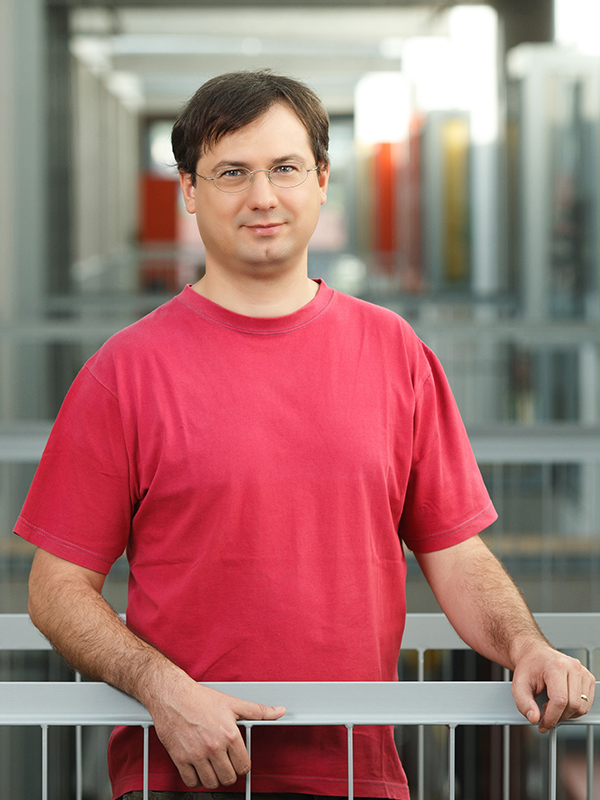• Course code:63280
• Credits:6
• Semester: summer
• Contents

Lectures:

1. Intro: about methods of algorithm design, analysis of algorithms, and  computational complexity of algorithms and problems
2. Divide-and-Conquer: description of  the method, examples of problems and algorithms (see examples 12 below)
3. Greedy  method: description, examples
4. Iterative improvement: descr., examples
5. Dynamic programming: descr., examples
6. Backtracking: description, examples
7. Branch&Bound: description, examples
8. Linear programming: descr., Simplex algorithm, examples
10. NP-hard computational problems: lower bounds on time complexity, informally about P, NP and NP-hard problems;
11. Methods of solving NP-hard problems:   heuristic algorithms,  approximation algorithms, randomized algorithms,  parameterized algorithms, exact exponential algorithms, examples
12. Example  problems and algorithms: advanced sorting & Heapsort, Quicksort;     selection problem & linear algorithms;        matrix multiplication & Strassen alg.; Discrete Fourier Transformation & FFT alg; string matching &  Knuth-Morris-Pratt;  elementary and other graph problems and algorithms (searching a graph; topological sort; maximum flow & Ford-Fulkerson alg.; shortest paths &  algorithms of  Bellman-Ford,  and  Floyd-Warshall); selected problems from computational geometry.

Tutorial:  Students will  use the topics given during the lectures to independently solve practical problems (with the assistance of the TAs if needed).  They will implement several smaller programs (home works) as well as larger programs (seminars), and present them at the tutorial.

• Study programmes
• Distribution of hours per semester
45
hours
lectures
30
hours
laboratory work
• ProfessorInstructor
Room:R2.05 - Kabinet
TB
Teaching AssistantTeaching Assistant
Room:R2.30 - Laboratorij LTPOTeaching Assistant
Room:R2.61 - Kabinet
PP
Teaching Assistant